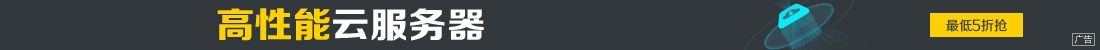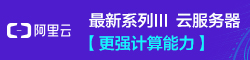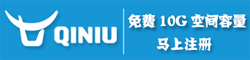# python生成随机红包的实例写法

••```def redbags(money, num=10):
import random
choice = random.sample(range(1, money * 100), num - 1)
choice.extend([0,money*100])
choice.sort()
return [(choice[i + 1] - choice[i]) / 100 for i in range(num)]```

```#!/usr/bin/env python
# -*-coding:utf-8 -*-

import random

dic={}
lis = ['KeLan','Monkey','Dexter','Superman','Iron Man','Robin']

def redpacket(cash,person,index):
if cash>0 and person !=1:
n = round(random.uniform(0.01,cash-(0.01*person)),2)
dic[lis[index]] = n
print str(n).ljust(4,"0")
person-=1
cash-=n
index+=1
redpacket(cash,person,index)
else:
dic[lis[index]]=round(cash,2)
print str(cash).ljust(4,"0")

redpacket(10,len(lis),0)
print dic
print "手气最佳:",max(dic.items(),key=lambda x:x)```